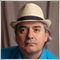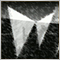Calculating on Range161

Hi, I´m having a problem calculation range bars. What I want is that it calculates a certain number of bars and wait for the next same number of bars to recalculate. Everytime I´m trying, when a new bar come in it reads the bars all over again and stays dinamically instead of fixed.

For exemple, calculate highest bar Starting on 0 and through 10, that I´ve got it. But now I want the indicator to wait 10 more bars to recalculate. What´s happening is that it calculate the firsts 10 bars, when a newbar comes it calculate when next 10 instead of letting the array 1 - 11 fixed and wait for another 10...

Sorry about my english, I don´t know if I was clear enough.4946

Leonardo Guarda: Hi, I´m having a problem calculation range bars. What I want is that it calculates a certain number of bars and wait for the next same number of bars to recalculate. Everytime I´m trying, when a new bar come in it reads the bars all over again and stays dinamically instead of fixed. For exemple, calculate highest bar Starting on 0 and through 10, that I´ve got it. But now I want the indicator to wait 10 more bars to recalculate. What´s happening is that it calculate the firsts 10 bars, when a newbar comes it calculate when next 10 instead of letting the array 1 - 11 fixed and wait for another 10...

Then show your code. We cannot possible "guess" what you are doing wrong or right until we understand the context of your code.

Also state, if this MQL4 or MQL5, whether it is an Indicator, EA or Script.77659

Leonardo Guarda:

Hi, I´m having a problem calculation range bars. What I want is that it calculates a certain number of bars and wait for the next same number of bars to recalculate. Everytime I´m trying, when a new bar come in it reads the bars all over again and stays dinamically instead of fixed.

For exemple, calculate highest bar Starting on 0 and through 10, that I´ve got it. But now I want the indicator to wait 10 more bars to recalculate. What´s happening is that it calculate the firsts 10 bars, when a newbar comes it calculate when next 10 instead of letting the array 1 - 11 fixed and wait for another 10...

Sorry about my english, I don´t know if I was clear enough.

it isn't clear too much for me but as i understand  you can make and integer let name it as bars in general

int bars;

now in oninit() function let it equals bars on chart:

bars=Bars;

and in ontick() function relate bars numbers to what you need like this:

if(Bars>bars+10)

{

//"what you need here";

bars=Bars;  //to start calc again after your code runs

}161

Abdullah Alrai:

it isn't clear too much for me but as i understand  you can make and integer let name it as bars in general

now in oninit() function let it equals bars on chart:

and in ontick() function relate bars numbers to what you need like this:

Tyvm, I´ve got it solved...